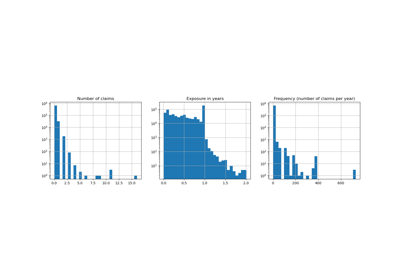# sklearn.dummy.DummyRegressor¶

class sklearn.dummy.DummyRegressor(*, strategy='mean', constant=None, quantile=None)[source]

Regressor that makes predictions using simple rules.

This regressor is useful as a simple baseline to compare with other (real) regressors. Do not use it for real problems.

Read more in the User Guide.

New in version 0.13.

Parameters:
strategy{“mean”, “median”, “quantile”, “constant”}, default=”mean”

Strategy to use to generate predictions.

• “mean”: always predicts the mean of the training set

• “median”: always predicts the median of the training set

• “quantile”: always predicts a specified quantile of the training set, provided with the quantile parameter.

• “constant”: always predicts a constant value that is provided by the user.

constantint or float or array-like of shape (n_outputs,), default=None

The explicit constant as predicted by the “constant” strategy. This parameter is useful only for the “constant” strategy.

quantilefloat in [0.0, 1.0], default=None

The quantile to predict using the “quantile” strategy. A quantile of 0.5 corresponds to the median, while 0.0 to the minimum and 1.0 to the maximum.

Attributes:
constant_ndarray of shape (1, n_outputs)

Mean or median or quantile of the training targets or constant value given by the user.

n_features_in_None

DEPRECATED: n_features_in_ is deprecated in 1.0 and will be removed in 1.2.

n_outputs_int

Number of outputs.

DummyClassifier

Classifier that makes predictions using simple rules.

Examples

>>> import numpy as np
>>> from sklearn.dummy import DummyRegressor
>>> X = np.array([1.0, 2.0, 3.0, 4.0])
>>> y = np.array([2.0, 3.0, 5.0, 10.0])
>>> dummy_regr = DummyRegressor(strategy="mean")
>>> dummy_regr.fit(X, y)
DummyRegressor()
>>> dummy_regr.predict(X)
array([5., 5., 5., 5.])
>>> dummy_regr.score(X, y)
0.0


Methods

 fit(X, y[, sample_weight]) Fit the random regressor. get_params([deep]) Get parameters for this estimator. predict(X[, return_std]) Perform classification on test vectors X. score(X, y[, sample_weight]) Return the coefficient of determination R^2 of the prediction. set_params(**params) Set the parameters of this estimator.
fit(X, y, sample_weight=None)[source]

Fit the random regressor.

Parameters:
Xarray-like of shape (n_samples, n_features)

Training data.

yarray-like of shape (n_samples,) or (n_samples, n_outputs)

Target values.

sample_weightarray-like of shape (n_samples,), default=None

Sample weights.

Returns:
selfobject

Fitted estimator.

get_params(deep=True)[source]

Get parameters for this estimator.

Parameters:
deepbool, default=True

If True, will return the parameters for this estimator and contained subobjects that are estimators.

Returns:
paramsdict

Parameter names mapped to their values.

property n_features_in_

DEPRECATED: n_features_in_ is deprecated in 1.0 and will be removed in 1.2.

predict(X, return_std=False)[source]

Perform classification on test vectors X.

Parameters:
Xarray-like of shape (n_samples, n_features)

Test data.

return_stdbool, default=False

Whether to return the standard deviation of posterior prediction. All zeros in this case.

New in version 0.20.

Returns:
yarray-like of shape (n_samples,) or (n_samples, n_outputs)

Predicted target values for X.

y_stdarray-like of shape (n_samples,) or (n_samples, n_outputs)

Standard deviation of predictive distribution of query points.

score(X, y, sample_weight=None)[source]

Return the coefficient of determination R^2 of the prediction.

The coefficient R^2 is defined as (1 - u/v), where u is the residual sum of squares ((y_true - y_pred) ** 2).sum() and v is the total sum of squares ((y_true - y_true.mean()) ** 2).sum(). The best possible score is 1.0 and it can be negative (because the model can be arbitrarily worse). A constant model that always predicts the expected value of y, disregarding the input features, would get a R^2 score of 0.0.

Parameters:
XNone or array-like of shape (n_samples, n_features)

Test samples. Passing None as test samples gives the same result as passing real test samples, since DummyRegressor operates independently of the sampled observations.

yarray-like of shape (n_samples,) or (n_samples, n_outputs)

True values for X.

sample_weightarray-like of shape (n_samples,), default=None

Sample weights.

Returns:
scorefloat

R^2 of self.predict(X) wrt. y.

set_params(**params)[source]

Set the parameters of this estimator.

The method works on simple estimators as well as on nested objects (such as Pipeline). The latter have parameters of the form <component>__<parameter> so that it’s possible to update each component of a nested object.

Parameters:
**paramsdict

Estimator parameters.

Returns:
selfestimator instance

Estimator instance.

## Examples using sklearn.dummy.DummyRegressor¶Poisson regression and non-normal loss

Poisson regression and non-normal loss